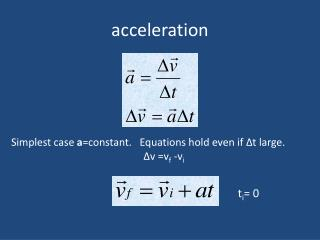DownloadDownload Presentationacceleration

# acceleration

Télécharger la présentation## acceleration

- - - - - - - - - - - - - - - - - - - - - - - - - - - E N D - - - - - - - - - - - - - - - - - - - - - - - - - - -
##### Presentation Transcript

1. acceleration Simplest case a=constant. Equations hold even if Δt large. Δv =vf -vi ti= 0

2. Example : If a car traveling at 28 m/s is brought to a full stop 4.0 s after the brakes are applied, find the average acceleration during braking. Given: vi = +28 m/s, vf = 0 m/s, and t = 4.0 s.

3. If a = const. Not true in general If a = const. one dimensional motion

4. Fig. 04.01 Vav gives same area Hence same distance

5. Constant acceleration Δx= vavt Δx= = vit+1/2 at2 ti = 0

6. Fig. 04.02

7. Δx=vxΔt=Area= viΔt (blue area)+½ Δt (aΔt) (gold area) = viΔt + ½ aΔt2

8. a=constant • Δx=vavΔt = 1/2(vi+vf)Δt but vf = vi + aΔt so Δt = (vf-vi)/a Δx = 1/2(vi+vf)Δt = 1/2 (vi+vf) (vf-vi)/a • = 1/(2a) (vf2-vi2) = Δx • (vf2-vi2) =2aΔx

9. Plotted vs time

10. Minimum length of runway A fully loaded 747 with all engines at full throttle accelerates at 2.6 m/s2. Its minimum takeoff speed is 70 m/s. How long will it require to reach take off speed? What is the minimum length of a runway for a 747. vf = vi + aΔt (vf2-vi2) =2aΔx

11. Problem • A car traveling at a speed of 30 m/s. A deer runs across the road and the driver slams on the brakes. It takes .75 s to begin applying the brakes. With the brakes on the car decelerates at 6 m/s2. How far does the car travel from the instant the driver sees the deer until he stops.

12. Free Fall • All objects, under the influence of only gravity fall. (We are neglecting air resistance) • They all fall with a constant acceleration (down) of • g = 9.8 m/s2 • The mass of the object doesn’t matter! Heavy and light objects all fall with the same g • It doesn’t matter in which direction it is moving it has an acceleration of g • Since we normally take y + up free fall is -g

13. Free Fall Slide 2-36

14. You drop a stone off a cliff and hear it hit the ground after two seconds. How high is the cliff? • This is an how far question • Δx=1/2 at2 • Substitute the numbers a=9.8 t=2 • Δx=19.6 • How fast is it going when it hits?

15. Example Problem Tennis balls are tested by measuring their bounce when dropped from a height of approximately 2.5 m. What is the final speed of a ball dropped from this height? Slide 2-34

16. Throwing stones (up) • What happens if you toss a stone straight up? v(3) v(1) v(0) .v(4) =0. It reaches its highest point when it stops going up, i.e. when v = 0 a is always downward it is g

17. Throwing stones (up) • What happens when it starts coming down again? v(3) v(1) v(0) .v(4) =0. It reaches its highest point when it stops going up and then begins to fall with a=-g. It re-traces its path and velocity but down a is always downward it is g

18. Checking Understanding An arrow is launched vertically upward. It moves straight up to a maximum height, then falls to the ground. The trajectory of the arrow is noted. Which choice below best represents the arrow’s acceleration at the different points? A  E  B  D; C  0 E  D  C  B  A A  B  C  D  E A  B  D  E; C  0 Slide 2-37

19. Answer An arrow is launched vertically upward. It moves straight up to a maximum height, then falls to the ground. The trajectory of the arrow is noted. Which choice below best represents the arrow’s acceleration at the different points? A  E  B  D; C  0 E  D  C  B  A A  B  C  D  E A  B  D  E; C  0 Slide 2-38

20. Checking Understanding An arrow is launched vertically upward. It moves straight up to a maximum height, then falls to the ground. The trajectory of the arrow is noted. Which graph best represents the vertical velocity of the arrow as a function of time? Ignore air resistance; the only force acting is gravity. Slide 2-39

21. Answer An arrow is launched vertically upward. It moves straight up to a maximum height, then falls to the ground. The trajectory of the arrow is noted. Which graph best represents the vertical velocity of the arrow as a function of time? Ignore air resistance; the only force acting is gravity. D. Slide 2-40

22. y viy x ay Example: You throw a ball into the air with speed 15.0 m/s; how high does the ball rise? Given: viy = +15.0 m/s; ay = 9.8 m/s2

23. Also an how far question • (vf2-vi2) =2ad • What is vf? vi? What is a? (be careful of signs!)

24. You toss a ball straight up with an initial vi=25m/s. You then become distracted. How long until the ball clunks you on your head?

25. y x 369 m Example : A penny is dropped from the observation deck of the Empire State Building 369 m above the ground. With what velocity does it strike the ground? Ignore air resistance. How long will it take to hit? Given: viy = 0 m/s, ay =  9.8 m/s2, y = 369 m ay Unknown: vyf